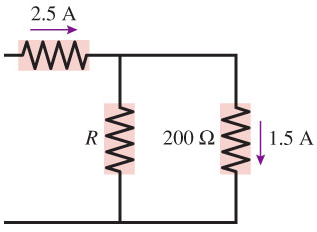# Problem: The currents in two resistors in a circuit are shown in the figure above. What is the value of resistor R?

###### FREE Expert Solution

Ohm's law:

$\overline{){\mathbf{V}}{\mathbf{=}}{\mathbf{i}}{\mathbf{R}}}$

From Kirchhoff's junction rule:

2.5 + iR = 1.5

iR = 1.5 - 2.5 = -1 A = 1 A

87% (212 ratings)###### Problem Details

The currents in two resistors in a circuit are shown in the figure above. What is the value of resistor R?